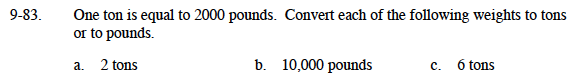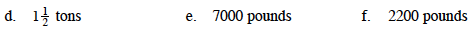### Home > MC1 > Chapter 9 > Lesson 9.2.3 > Problem9-83

9-83.2 tons is twice as heavy as 1 ton. If 1 tons is the same as 2000 pounds, how many pounds would be twice as heavy?

$\frac{10,000\text{ pounds}}{2000\text{ pounds in each ton}}$

12,000 poundsHalf of one ton is half of 2,000 pounds.

$3\frac{1}{2}\text{ tons}$

$\frac{2200 \text{ pounds}}{2000\text{ pounds}}$